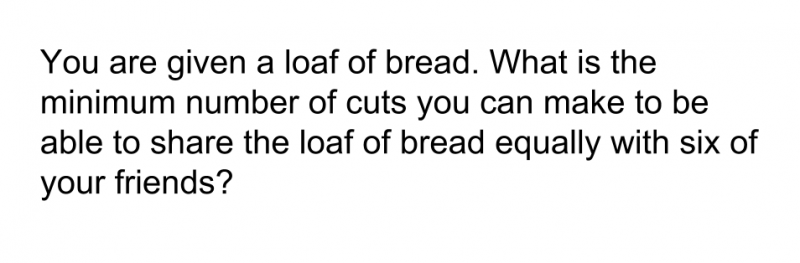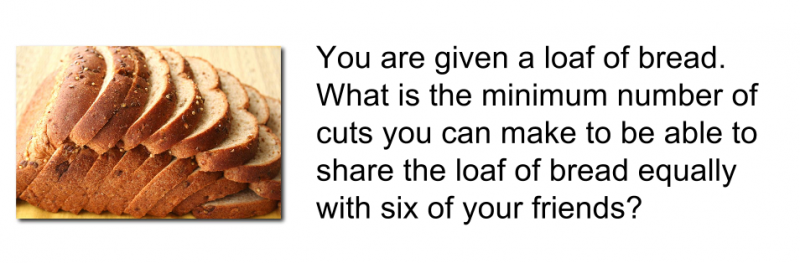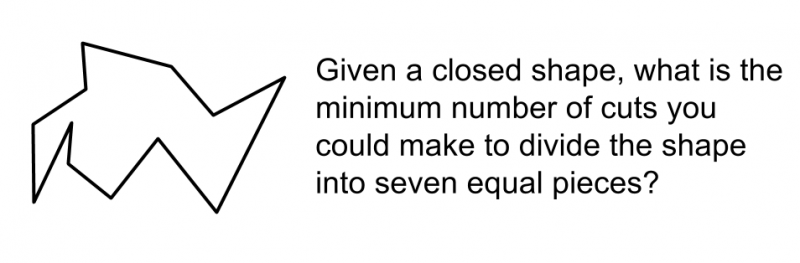This is continued from here.

Imagine a scale for the open-endedness of a mathematical task.At one of the scale you learn a lot about what exactly kids can and cannot do but you sacrifice the opportunity for students to learn much from the activity. At the other end of the scale, you have no idea what children know, but you gain the potential for students to learn a tremendous amount. At one end of the scale, children know exactly what they are supposed to do, but at the other end of the scale, they may have no idea how to get started, and in doing so, learn very little as they struggle.

An ideal mathematics task for students is probably somewhere in the middle. A long term goal for any mathematics class should be to help students be able to cope with more ambigious mathematics activities than they were able to do before.

Try this mathematics problem, then continue reading.Another issue that comes up with designing mathematics tasks is the hidden assumptions people make when you give them problems. When you read that problem, how did you imagine that loaf of bread in your head? How did you define the word cut? How many people are sharing the bread? The answers to these three questions will determine to a large extent what your solution to this problem looks like. This means that when designing mathematical tasks, we not only have to consider how we have written the task, we also need to consider how students may read the task, and what assumptions they are likely to make.Context clues also matter. Notice how this version of the problem adds a pretty large context clue. What differences does this make in how students respond to the task?

Now look at this variation in the task.Technically, this question is the same mathematical problem as the first two, but it will end up with a much wider variation in responses than either of the first two problems will. All we have changed is the context the student is presented with when they are given the problem.

This leads us to two methods for changing the open-endedness of a mathematical task.

1. Change the context of the problem to make the context more abstract.
2. Change the inherent assumptions in the problem, or at least point out that those assumptions exist.

In a future post, I will explore more ways to consider the open-endedness of a mathematical task, and offer some suggestions on ways to change standard mathematical tasks, while still giving students sufficient scaffolding to know where to begin.